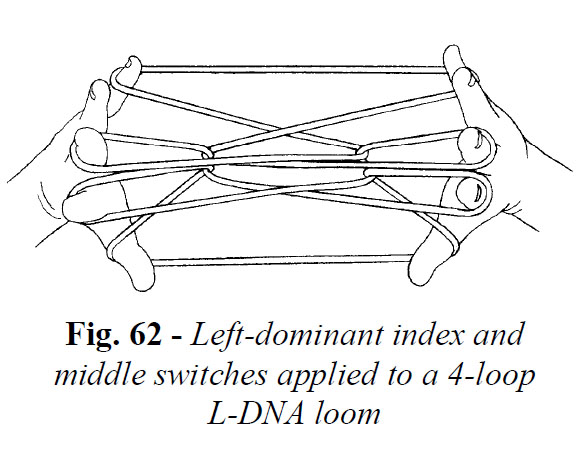# openings and dominant switches

OOPS! i made a mistake when i compared the inuit hole made from a left dominant switch applied to an ldna loom and a left dominant switch applied to an opening A loom (r l). i thought the figures were different. they are not, although the positions just before ending the figures with the inuit net are different. by joining the loops into the double center circles, this difference is nullified!

DOMINANT SWITCHES

Loop exchanges are often used to modify looms and add richness to designs.  The figure described on page 244 is a good example. After forming the loom (Opening A), the right and left index loops are exchanged. As a result, the near (and far) index strings of the loom interlock rather than merely cross (fig. 58). In loop exchanges order is important. If one starts with Opening A (right palmar string picked up first), the left index
loop must pass over the right index loop during the exchange (i call this a left-dominant exchange).  If done vice-versa, the loom dissolves.Traditional loop exchanges (fingers inserted from above during the transfers) cannot be applied to DNA looms: the looms will partially dissolve, regardless of the order in which the loops are exchanged. For this reason i invented a new type of exchange, which i call a switch. In a switch exchange, the fingers are inserted from below during the loop transfers. Again, order is important. One can define two types of switches: a left-dominant switch (left loop passes over right loop) and a right-dominant switch (right loop passes over left loop). To practice, set up a 3-loop L-DNA loom and proceed as follows:

Left-Dominant Index Switch

• Transfer the left index loop to the top of the right index, inserting the right index from below and from the near side; pass the left index down through the right upper index loop, then insert it, from below and from the near side, into the right lower index loop; lift this loop off the right index, drawing it up through the right upper index loop as you return the left index to its original position.The result is shown in fig. 59. The effect of applying a left-dominant index switch to an L-DNA loom is magical: the far thumb-far index string of one hand interlocks with the near index-near little finger string of the opposite hand, As a result, a small hole forms in the center of the loom.

One can immediately design a simple figure that showcases this feature:

• 3-loop L-DNA loom
• Left-dominant index switch
• Inuit Out, Power Lift (fig. 60).A right dominant index switch is similarly defined. To practice, set up a 3-loop R-DNA loom and proceed as follows:

Right Dominant Index Switch

• Transfer the right index loop to the top of the left index, inserting the left index from below and from the near side; pass the right index down through the left upper index loop, then insert it, from below and from the near side, into the left lower index loop; lift this loop off the left index, drawing it up through the left upper index loop as you return the right index to its original position.

The result is shown in fig. 61.Note that going “against the grain” of a loom (applying a left dominant switch
to a right DNA loom) destroys the symmetry of the central design motif and often results in a tangled mass of strings (which can be exploited as shown in the figures below.the top image is the r l r l false face, the bottom is the lrlr false faceDominant switches can also be applied to 4- and 5-loop DNA looms. In a 4-loop DNA loom both the index and middle finger loops can be switched to give two nested holes in the center of the loom (fig. 62).In a 5-loop DNA loom, the index, middle, and ring finger loops can all be switched to give three nested holes.

To investigate the effects of introducing dominant switches into the looms of 4- and 5-loop tennis nets, i devised two simple test patterns (fig 63, chart 39, and fig. 64, chart 40). In my circle notation charts, an “LS” placed under a circle indicates that a left dominant switch should be applied to the corresponding loop (“RS” stands for right-dominant switch):